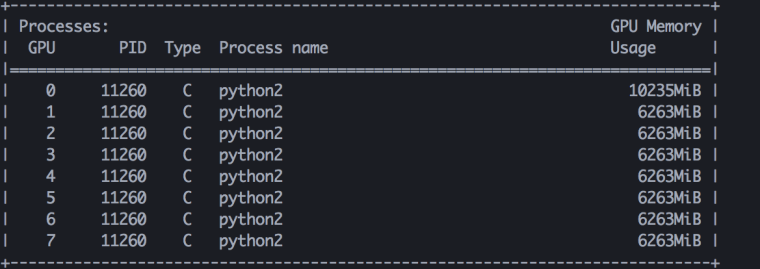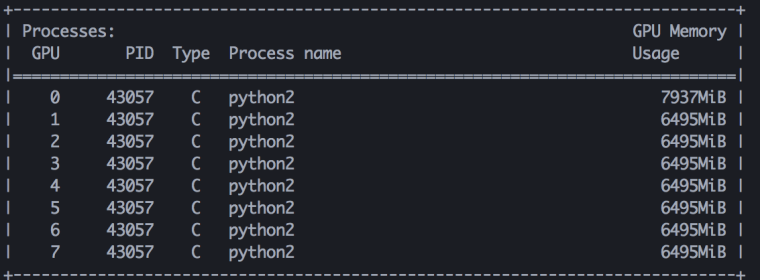bindog study Machine Learning & Security

# 显存均衡的模型并行(PyTorch实现)

2019-09-05

## 0x01 朴素的模型并行

class FullyConnected(nn.Module):
def __init__(self, in_dim, out_dim, num_gpu, model_parallel=False):
super(FullyConnected, self).__init__()
self.num_gpu = num_gpu
self.model_parallel = model_parallel
if model_parallel:
self.fc_chunks = nn.ModuleList()
for i in range(num_gpu):
_class_num = out_dim // num_gpu
if i < (out_dim % num_gpu):
_class_num += 1
self.fc_chunks.append(
nn.Linear(in_dim,
_class_num,
bias=False).cuda(i)
)
else:
self.classifier = nn.Linear(
in_dim,
out_dim,
bias=False)

def forward(self, x):
if self.model_parallel:
x_list = []
for i in range(self.num_gpu):
_x = self.fc_chunks[i](x.cuda(i))  # 分别在不同的卡上计算
x_list.append(_x)
x = torch.cat(x_list, dim=1)  # 把结果concat起来
return x
else:
return self.classifier(x)## 0x02 显存均衡的模型并行

### 计算softmax

def stable_softmax(x):
z = x - max(x)
numerator = np.exp(z)
denominator = np.sum(numerator)
softmax = numerator / denominator
return softmax


### 反向传播

class ModelParallelCrossEntropy(nn.Module):
def __init__(self):
super(ModelParallelCrossEntropy, self).__init__()

# args is compute loss flag, args is label_tuple
# args[2:] is logit parts
def forward(self, *args):
return ModelParallelCrossEntropyFunc(args, args)(*args[2:])

class ModelParallelCrossEntropyFunc(Function):
def __init__(self, compute_loss, label_tuple):
self.batch_size = label_tuple.size()
self.compute_loss = compute_loss
self.label_split = label_tuple

def forward(self, *args):  # args is list of logit parts
# for numerical stability
max_list = []
for arg in args:
m, _ = torch.max(arg, dim=1, keepdim=True)
max_list.append(m)
mc = torch.cat(max_list, dim=1)
m, _ = torch.max(mc, dim=1, keepdim=True)
nargs = [arg - m.to(gpu_id) for gpu_id, arg in enumerate(args)]

# get exp sum
exp_logit_list = []
exp_sum_list = []
for gpu_id, narg in enumerate(nargs):
exp_logit = torch.exp(narg)
exp_logit_list.append(exp_logit)
exp_sum = torch.sum(exp_logit, dim=1, keepdim=True)
exp_sum_list.append(exp_sum)

# compute softmax output
softmax_list = []
for gpu_id, narg in enumerate(nargs):
softmax = exp_logit_list[gpu_id] / exp_sum_all.to(gpu_id)
softmax_list.append(softmax)
# save the softmax output, we will need it in backward
self.save_for_backward(*softmax_list)

loss = torch.zeros(1)
if self.compute_loss:
_loss_list = []
for gpu_id, softmax in enumerate(softmax_list):
_loss = torch.sum(softmax * self.label_split[gpu_id], dim=1)
_loss_list.append(_loss)
log_loss = -torch.log(_loss)
loss = torch.mean(log_loss)

return loss

for gpu_id, softmax in enumerate(self.saved_variables):
grad_logit = (softmax - self.label_split[gpu_id]) / self.batch_size## 0x03 和其他魔改loss相结合

class FullyConnected_AM(nn.Module):
def __init__(self, in_dim, out_dim, num_gpus=1, model_parallel=False, class_split=None, margin=0.35, scale=30):
super(FullyConnected_AM, self).__init__()
self.num_gpus = num_gpus
self.model_parallel = model_parallel
if self.model_parallel:
self.am_branches = nn.ModuleList()
for i in range(num_gpus):
self.am_branches.append(AM_Branch(in_dim, class_split[i], margin, scale).cuda(i))
else:
self.am = AM_Branch(in_dim, out_dim, margin, scale)

# 非模型并行情况下，labels为one-hot形式
# 模型并行情况下，labels是一个list，里面每个元素是labels的one-hot形式的一小部分(按class方向划分、且已经在对应的显卡上)，concat起来就是完整的one-hot形式label
def forward(self, x, labels=None):
if self.model_parallel:
output_list = []
for i in range(self.num_gpus):
output = self.am_branches[i](x.cuda(i), labels[i])
output_list.append(output)
return tuple(output_list)
else:
return self.am(x, labels)

class AM_Branch(nn.Module):
def __init__(self, in_dim, out_dim, margin=0.35, scale=30):
super(AM_Branch, self).__init__()
self.m = margin
self.s = scale
#  training parameter
self.weight.data.uniform_(-1, 1).renorm_(2, 1, 1e-5).mul_(1e5)

# 这里的label必须是已经转换为one-hot形式
# 如果是模型并行下，label是one-hot形式的一部分，且位于对应的显卡上
def forward(self, x, label):
x_norm = x.pow(2).sum(1).pow(0.5)
w_norm = self.weight.pow(2).sum(0).pow(0.5)
cos_theta = torch.mm(x, self.weight) / x_norm.view(-1, 1) / w_norm.view(1, -1)
cos_theta = cos_theta.clamp(-1, 1)
phi = cos_theta - self.m

index = label.data
index = index.byte()

output = cos_theta * 1.0
output[index] = phi[index]
output *= self.s

return output


## 0x04 说明

python train.py --gpus=0,1,2,3 --data_path=/your/data/path --num_classes=3000000 --am --model_parallel